# How to Calculate and Solve for Solidification Time | Solidification of Metals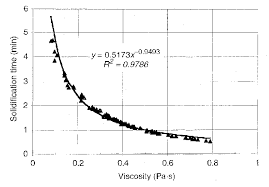The image above represents solidification time.

To compute for solidification time, three essential parameters are needed and these parameters are Chvorinov’s Constant (C), Volume (V) and Surface Area (A).

The formula for calculating solidification time:

t = C(V / A)

Where:

t = Solidification Time
C = Chvorinov’s Constant
V = Volume
A = Surface Area

Let’s solve an example;
Find the solidification time when the chvorinov’s constant is 15, the volume is 12 and the surface area is 8.

This implies that;

C = Chvorinov’s Constant = 15
V = Volume = 12
A = Surface Area = 8

t = C(V / A)
t = 15(12 / 8)
t = 15(1.5)
t = 22.5

Therefore, the solidification time is 22.5s.

Calculating the Chvorinov’s Constant when the Solidification Time, the Volume and the Surface Area is Given.

C = tA / V

Where:

C = Chvorinov’s Constant
t = Solidification Time
V = Volume
A = Surface Area

Let’s solve an example;
Find the Chvorinov’s Constant when the solidification time is 20, the volume is 12 and the surface area is 8.

This implies that;

t = Solidification Time = 20
V = Volume = 12
A = Surface Area = 8

C = tA / V
C = (20)(8) / 12
C = 160 / 12
C = 13.3

Therefore, the chvorinov’s constant is 13.3

Calculating the Volume when the Solidification Time, the Chvorinov’s Constant and the Surface Area is Given.

V = tA / C

Where:

V = Volume
t = Solidification Time
C = Chvorinov’s Constant
A = Surface Area

Let’s solve an example;
Find the volume when the solidification time is 10, the chvorinov’s constant is 4 and the surface area is 14.

This implies that;

t = Solidification Time = 10
C = Chvorinov’s Constant = 4
A = Surface Area = 14

V = tA / C
V = (10)(14) / 4
V = 140 / 4
V = 35

Therefore, the volume is 35.

Calculating the Surface Area when the Solidification Time, the Chvorinov’s Constant and the Volume is Given.

A = CV / t

Where:

A = Surface Area
t = Solidification Time
C = Chvorinov’s Constant
V = Volume

Let’s solve an example;
Find the surface area when the solidification time is 18, the chvorinov’s constant is 24 and the volume is 12.

This implies that;

t = Solidification Time = 18
C = Chvorinov’s Constant = 24
V = Volume = 12

A = CV / t
A = (24)(12) / 18
A = 288 / 18
A = 16

Therefore, the surface area is 16.

Nickzom Calculator – The Calculator Encyclopedia is capable of calculating the solidification time.

To get the answer and workings of the solidification time using the Nickzom Calculator – The Calculator Encyclopedia. First, you need to obtain the app.

You can get this app via any of these means:

To get access to the professional version via web, you need to register and subscribe for NGN 2,000 per annum to have utter access to all functionalities.
You can also try the demo version via https://www.nickzom.org/calculator

Apple (Paid) – https://itunes.apple.com/us/app/nickzom-calculator/id1331162702?mt=8
Once, you have obtained the calculator encyclopedia app, proceed to the Calculator Map, then click on Materials and Metallurgical under Engineering.Now, Click on Solidification of Metal under Materials and Metallurgical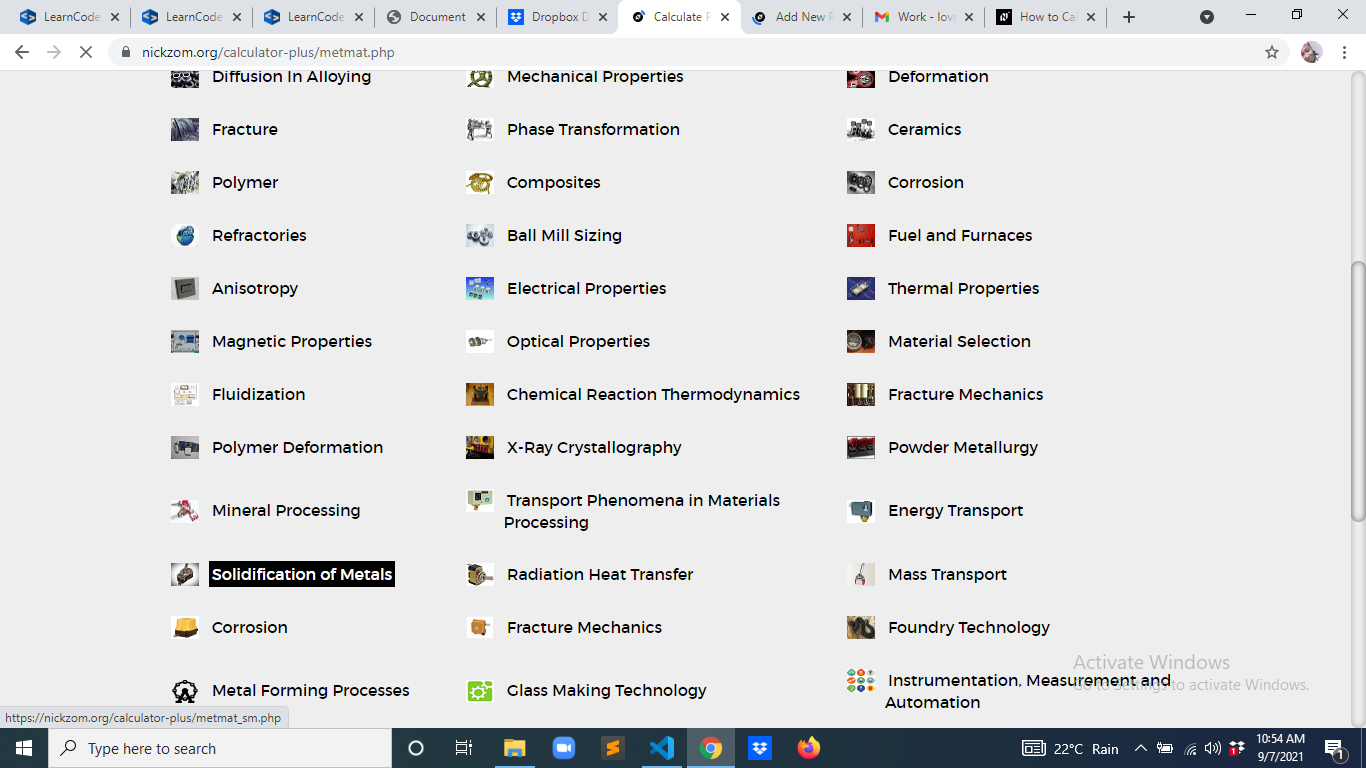Now, Click on Solidification Time under Solidification of Metal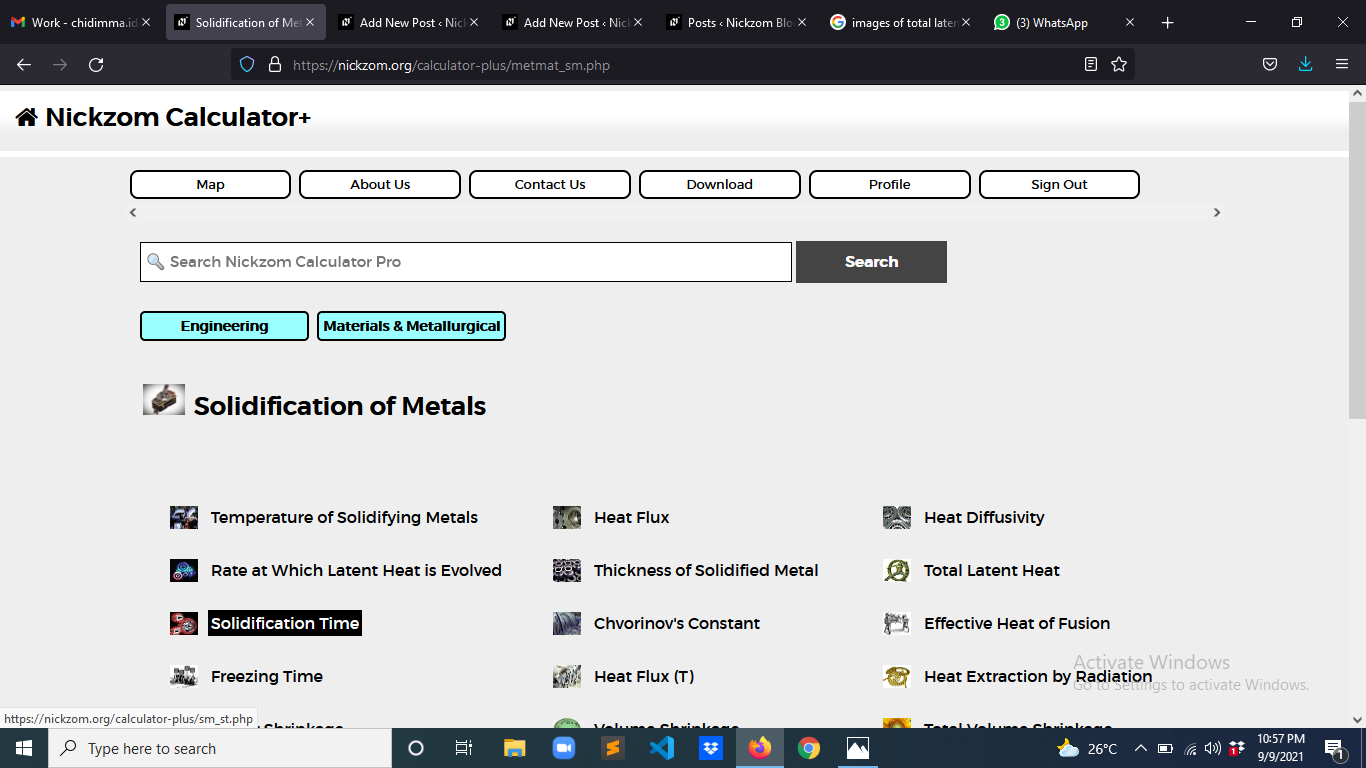The screenshot below displays the page or activity to enter your values, to get the answer for the solidification time according to the respective parameter which is the Chvorinov’s Constant (C), Volume (V) and Surface Area (A).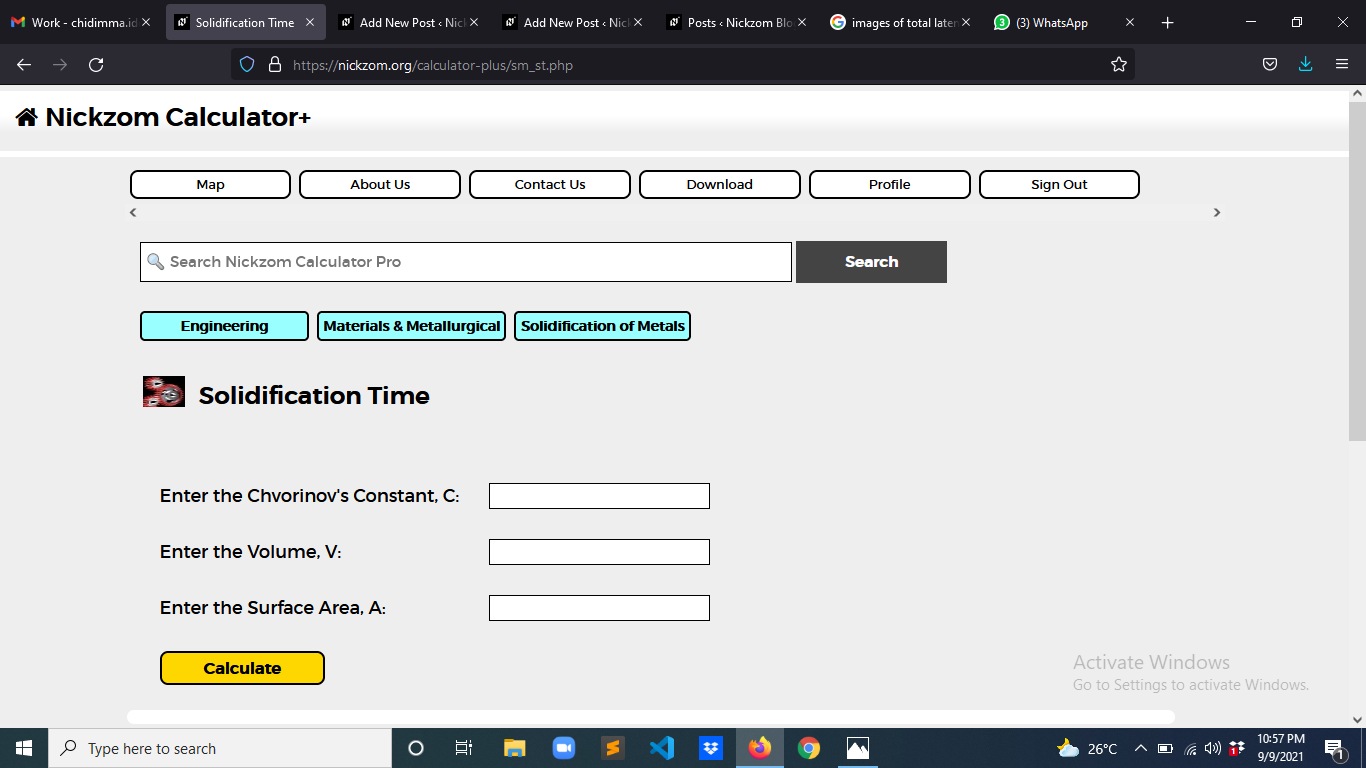Now, enter the values appropriately and accordingly for the parameters as required by the Chvorinov’s Constant (C) is 15, Volume (V) is 12 and Surface Area (A) is 8.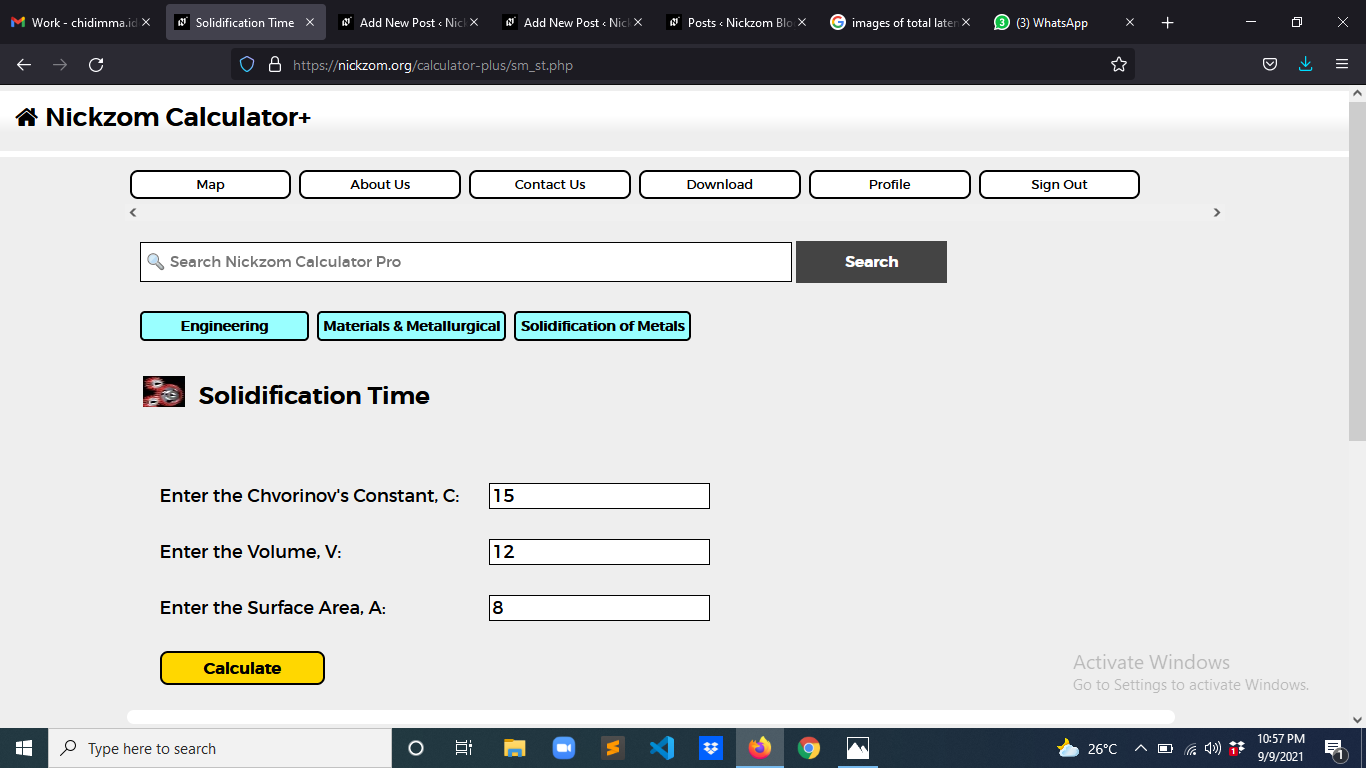Finally, Click on Calculate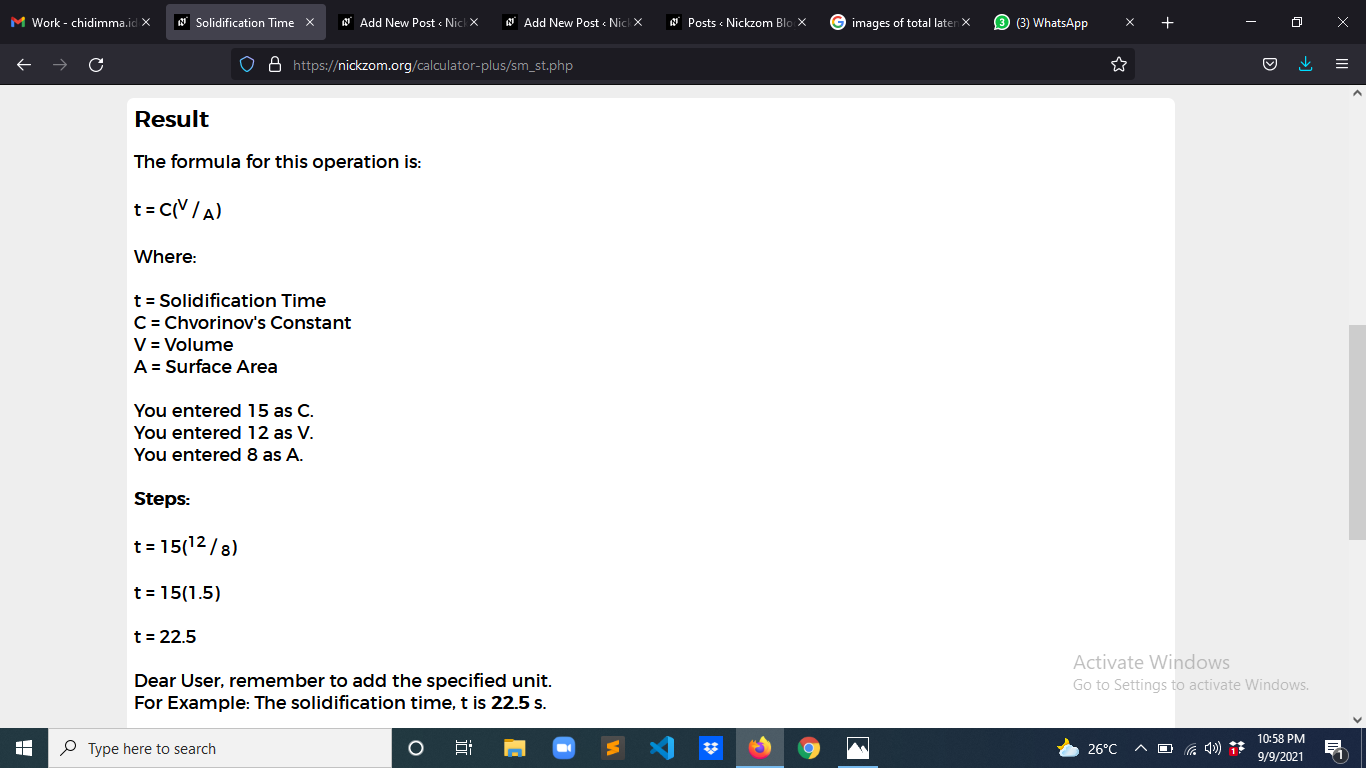As you can see from the screenshot above, Nickzom Calculator– The Calculator Encyclopedia solves for the solidification time and presents the formula, workings and steps too.